linear equations are presented. If a group of x and y values [or ordered pairs, P(x,y)] that satisfy a given linear equation are plotted on a coordinate system, the resulting graph is a straight line. When higher-ordered equations such as are encountered, the resulting graph is not a straight line. However, the points whose coordinates satisfy most of the equaŁtions in x and y are normally not scattered in a random field."> The Locus of an EquationCustom SearchTHE LOCUS OF AN EQUATION In chapter 1 of this course, methods for analysis of linear equations are presented. If a group of x and y values [or ordered pairs, P(x,y)] that satisfy a given linear equation are plotted on a coordinate system, the resulting graph is a straight line. When higher-ordered equations such as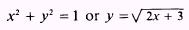are encountered, the resulting graph is not a straight line. However, the points whose coordinates satisfy most of the equaŁtions in x and y are normally not scattered in a random field. If the values are plotted, they will seem to follow a line or curve (or a combination of lines and curves). In many texts the plot of an equation is called a curve, even when it is a straight line. This curve is called the locus of the equation. The locus of an equation is a curve containing those points, and only those points, whose coordinates satisfy the equation. At times the curve may be defined by a set of conditions rather than by an equation, though an equation may be derived from the given conditions. Then the curve in question would be the locus of all points that fit the conditions. For instance a circle may be said to be the locus of all points in a plane that is a fixed distance from a fixed point. A straight line may be defined as the locus of all points in a plane equidistant from two fixed points. The method of expressing a set of conditions in analytical form gives an equation. Let us draw up a set of conditions and translate them into an equation. EXAMPLE: What is the equation of the curve that is the locus of all points equidistant from the two points (5,3) and (2,1)? SOLUTION: First, as shown in figure 2-2, choose some point having coordinates (x,y). Recall from chapter 1 of this course that the distance between this point and (2,1) is given byThe distance between point (x,y) and (5,3) is given byEquating these distances, since the point is to be equidistant from the two given points, we haveSquaring both sides, we have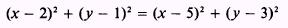Expanding, we haveCanceling and collecting terms, we see that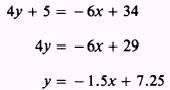This is the equation of a straight line with a slope of minus 1.5 and a y intercept of + 7.25.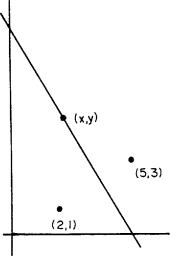Figure 2-2.-Locus of points equidisŁtant from two given points.   EXAMPLE: Find the equation of the curve that is the locus of all points equidistant from the line x = - 3 and the point (3,0). SOLUTION. As shown in figure 2-3, the distance from the point (x,y) on the curve to the line x = -3 isThe distance from the point (x,y) to the point (3,0) isEquating the two distances yields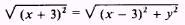Squaring and expanding both sides yieldsCanceling and collecting terms yields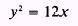which is the equation of a parabola.Figure 2-3.-Parabola. EXAMPLE: What is the equation of the curve that is the locus of all points in which the ratio of its distance from the point (3,0) to its distance from the line x = 25/3 is equal to 3/5? Refer to figure 2-4. SOLUTION: The distance from the point (x,y) to the point (3,0) is given by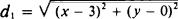The distance from the point (x,y) to the line x = 25/3 is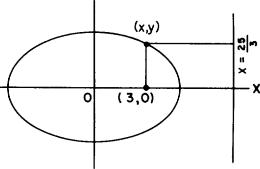Figure 2-4.-Ellipse. Since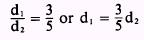thenSquaring both sides and expanding, we haveCollecting terms and transposing, we see thatDividing both sides by 16, we have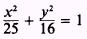This is the equation of an ellipse. PRACTICE PROBLEMS: Find the equation of the curve that is the locus of all points equidistant from the following: l. The points (0,0) and (5,4). 2. The points (3, - 2) and ( - 3,2). 3. The line x = - 4 and the point (3,4). 4. The point (4,5) and the line y = 5x - 4. HINT: Use the standard distance formula to find the distance from the point P(x,y) and the point P(4,5). Then use the formula for finding the distance from a point to a line, given in chapter 1 of this course, to find the distance from P(x,y) to the given line. Put the equation of the line in the form Ax + By + C=O. ANSWERS:Integrated Publishing, Inc. - A (SDVOSB) Service Disabled Veteran Owned Small Business